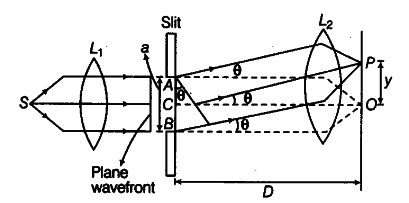# Using Huygens’ construction of secondary wavelets explain

(i) Using Huygens’ construction of secondary wavelets explain how a diffraction pattern is obtained on a screen due to a narrow slit on which a monochromatic beam of light is incident normally.
(ii) Show that the angular width of the first diffraction fringe is half that of the central fringe.
(iii) Explain why the maxima become weaker and weaker with increasing n.

(i)Consider a parallet beam of light from a lens falling on a slit AB.As, diffraction occurs, the pattern is focused on the screen with the help of lens . We will obtain a diffraction pattern that is central maximum at the centre O, flanked by a number of dark and bright fringes called secondary maxima and minima.
Each point on the plane wavefront AB sends out the secondary wavelets in all directions. The waves from points equidistant from the centre C , lying on the upper and lower half, reach point O with zero path difference and hence, reinforce each other producing maximum intensity at O.
(ii) Let,λ and a be the wavelength and slit width of diffracting system respectively. Let O be the position of central maximum.

(iii) On increasing the value of n, the part of slit contributing to the maximum decreases. Hence, the maximum becomes weaker.